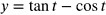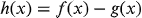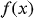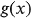1
71
views
2d
Problem

Problem 2d

Chapter 1: Functions and ModelsTextbook ExpertVerified Tutor
13 Oct 2021

Given information

The given function is.

Step-by-step explanation

Step 1.

This function is of the formwhereandare both trigonometric functions.The difference of trigonometric functions is itself a trigonometric function.So, this is a trigonometric function.